# Rubik's Cube Variants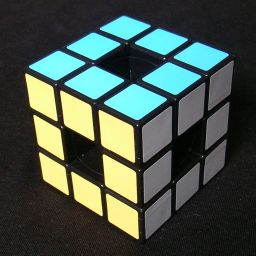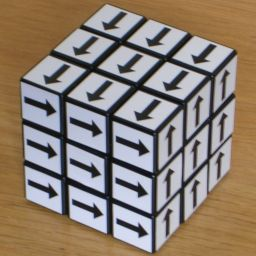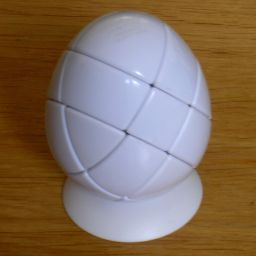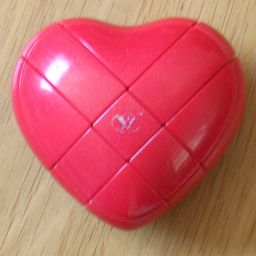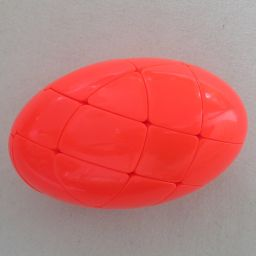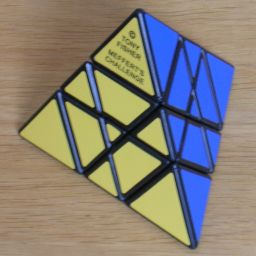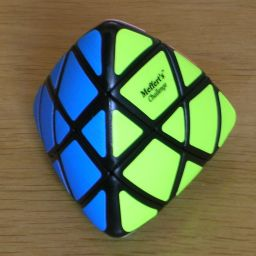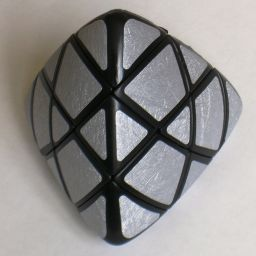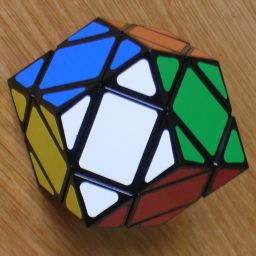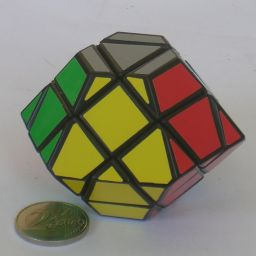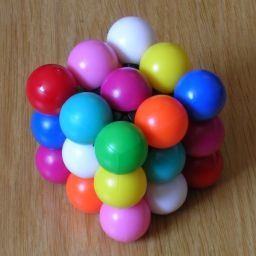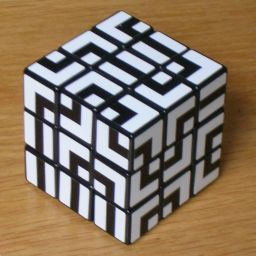Gathered together on this page is a slew of shape and colour variants of the 3×3×3 Rubik's Cube.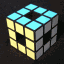The Void Cube has a cube variant that is missing its centres. It is equivalent to a normal Rubik's Cube from which the six face centre stickers have been removed. The Void Cube has a clever mechanism that allows it to have holes where the centres would be, holes that go all the way through the cube. It is as if the cube were made of just 12 edge cubies and 8 corner cubies stuck together.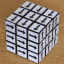Shepherd's Cube is a Rubik's Cube where all the stickers are the same and have an arrow on them. In its solved state, on every face the nine arrows must point in the same direction. The arrows on opposite faces point in opposite directions, and the pattern as a whole has rotational symmetry about one corner.
The original version made by Alistair Shepherd had heart-shaped stickers, but when David Singmaster reported on this variant in Cubic Circular 7&8 he illustrated it using arrows. It seems that since then the use of arrows in the design has become the standard.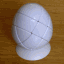The Egg Cube is a Rubik's Cube variant shaped like an egg. When mixed it changes shape. The pointy end of the egg is at a corner, so the solved egg has three-fold symmetry about that corner, similar to the Shepherd's Cube.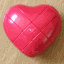The Heart Cube is another shape-changing Cube variant in the form of a heart. The pointy end of the heart is at an edge, so it has two-fold rotational symmetry about that edge.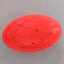The Oval Cube is a cheap Rubik's Cube variant shaped like an oval. Like the Heart Cube, it has an axis of two-fold rotational symmetry about an edge.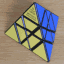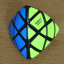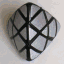The MasterMorphix is a Rubik's Cube variant shaped like a tetrahedron. There are versions with 4 colours, but more interestingly also ones with a single colour where only the shape matters.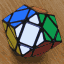The Rhombic Dodecahedron Cube is a Rubik's Cube variant shaped like a rhombic dodecahedron. The cube's edge pieces are now the centres of the rhomb-shaped faces, and so do not have visible orientation. It has six colours, and opposite faces have the same colour.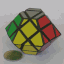The Flat Diamond Cube is a Rubik's Cube variant shaped like a hexagonal diamond. Two opposite corners have been flattened and are now the centres of hexagonal faces, and so do not have visible orientation. The rest of the cube has been cut to form 12 trapezium faces, but all other pieces are distinct and apart from the original cube's 6 face centres have visible orientation.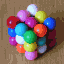The Nine-Colour Cube is a Rubik's Cube variant where each piece has just one of nine colours. The aim is to arrange it so that every face uses all nine colours exactly once. Six colours are used three times, once on a face centre, once on an edge, and on one corner. One colour occurs on two corners, and the remaining two colours each occur on three edges.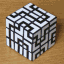The Labyrinth Cube is a Rubik's Cube variant designed by Christoph Lohe, where every facelet sticker has a line connecting two of its sides. The aim is to arrange it so that the lines join up, forming a single long loop that visits all 54 facelets. All the pieces are unique, but there are several solutions. Even if the cube is taken apart and put back together randomly, it is always solvable.
There are exactly 12 ways to put lines on an edge piece, so these all occur on the cube. There are 10 ways to make a corner piece, and the 8 patterns without rotational symmetry are used on the cube. Two face centres have straight lines on them (usually on adjacent faces) and four have bent lines.

Two cube variants have their own page on this site. They are:

There are many other shape and colour variants, such as ball-shaped ones, but most of them provide nothing new with regards to solving them, except sometimes orienting the face centres. The ones on this page are those which really are different, for example due to parity issues that do not occur on a normally coloured cube.

## The number of positions:

This table shows the calculation for the number of positions of each of these variants.
Corner permutations Corner Orientation Edge permutations Edge Orientation Centre Orientation Constraints Symmetry Positions
The Void Cube 8! *38 *12! *212 *16 /6 /24 = 3,604,333,606,207,488,000
Shepherd's Cube 8! / 3!2*3612*12! / 3!4*212 *46 /1 /8 = 632,859,672,969,216,000
The Egg Cube 8! / 3!2*3612*12! / 3!4*212 *46 /1 /1 = 5,062,877,383,753,728,000
The Heart Cube 8! / 2!4*38 *12! / 2!5*21012*46 /1 /1 = 1,038,048,078,587,756,544,000
The Oval Cube 8! / 2!4*38 *12! / 2!5*21012*46 /1 /1 = 1,038,048,078,587,756,544,000
MasterMorphix 1 colour 8! / 4!2*18 *1 *212 *26 /1 /2 = 9,175,040
MasterMorphix 4 colour 8! *38 *12! / 3!4*212 *46 /12 /1 = 136,697,689,361,350,656,000
Rhombic Dodecahedron Cube8! *38 *12! / 2!6*112 *46 /6 /1 = 1,351,625,102,327,808,000
Nine-Colour Cube 8! / 2! *18 *12! / 3!2*112 *16 /1 /1 = 268,240,896,000
Labyrinth Cube 8! *38 *12! *212 *46 /12 /1 =88,580,102,706,155,225,088,000

The constraints column is due to the usual twist and flip constraints when all edges and corners have visible orientation, and on the Mastermorphix the constraint that the corner permutation parity must be the same as the centre orientation parity. The symmetry column arises from the fact that, using only face turns and not middle slice turns, the solution can be constructed in different orientations. It accounts for the symmetry of the (possibly imaginary) central spider that hasn't yet been accounted for by the symmetry of the solution pattern itself.

## Notation:

Let the faces be denoted by the letters L, R, F, B, U and D (Left, Right Front, Back, Up and Down). Clockwise quarter turns of a face are denoted by the appropriate letter, anti-clockwise quarter turns by the letter with an apostrophe (i.e. L', R', F', B', U' or D'). Half turns are denoted by the letter followed by a 2 (i.e. L2, R2, F2, B2, U2 or D2). Turning a middle slice is shown using the subscript m, so for example Rm is a quarter turn of the middle slice between the R and L faces, clockwise when looking at the R face.

## Solutions:

All of these puzzles can obviously be solved using any Rubik's Cube solution, possibly augmented with a way to orient the face centres. Here are some useful sequences for orienting the face centres:

• U centre, 180 degrees: do R L U2 R' L' U twice
• U centre +90, R centre -90 degrees: do Rm' Um Rm U' Rm' Um' Rm U

Every quarter turn of a face is an odd permutation of the corners and of the edges. Therefore the parity of the corner or edge permutation shows whether an even or an odd number of quarter turns have been done. This means that it is not possible to twist a single face centre by a quarter turn without rearranging any other pieces - it must take an odd number of quarter turns to fix the centre, and so must cause an odd permutation on the edges and an odd permutation on the corners, and therefore at least one pair of edges must be swapped, as must at least one pair of corners.

Some of these variants have sets of identical corner pieces and of identical edge pieces. In that situation it is possible to invisibly swap those pieces and so cause a single face centre to twist a quarter turn in either direction. This can be a lot of work, so some useful move sequences will be given for those situations.

## The Void Cube:

There is one situation that occurs on the Void Cube that cannot happen on a normal cube, which is that you need to swap two pieces. This is an odd permutation and that is impossible on a cube without moving the centres. Conceptually, the simplest way to fix this parity is to do any middle slice move such as Rm and then solve it using no further middle slice moves or an even number of them. This is especially easy if you use a corners first solution method.

If you solve it by doing two layers first, and find that you need to swap two pieces in the last layer, then you can do this short parity fix: Rm2 U Rm U' Rm2. A longer sequence that just swaps the UF and UR edges is U F2 U' Rm' U F2 U2 Rm' U Rm.

## Shepherd's Cube and The Egg Cube:

These two puzzles have some identical edges and identical corners. It is therefore possible to invisibly apply an odd permutation on them and so twist a single face centre by a quarter turn seemingly without disturbing anything else. These two puzzles have an axis of three-fold rotational symmetry through a pair of opposite corners. To twist a face centre a quarter turn, hold the puzzle so that the face centre is in the U face, and a symmetric corner lies at the URF location, and then do D' F L' F R U R' F' L F2 D F.

## The Heart Cube and The Oval Cube:

Like Shepherd's Cube, these two puzzles also have some identical edges and identical corners. It is therefore possible to invisibly apply an odd permutation on them and so twist a single face centre by a quarter turn seemingly without disturbing anything else. These two puzzles have an axis of two-fold rotational symmetry through a pair of opposite edges. To twist a face centre a quarter turn, hold the puzzle so that the symmetric edges lies at the FL and BR locations, then do L' F2 R2 F' U R U2 R F2 L F to twist the centre of the U face.

## Mastermorphix:

The 4-colour Mastermorphix has 8 distinct corner pieces, by which I mean the corner pieces of the underlying cube, so the 4 tetrahedron corners and the 4 tetrahedron face centres. There is therefore no parity issue with the orientation of the six cube-face-centres (centres of the tetrahedron edges). A standard supercube solution will therefore also solve this puzzle without needing any parity fixes. You can however invisibly twist the tetrahedron face centres, so it is possible to seemingly twist just a single tetrahedron corner piece. You can also apparently flip edges by swapping identical pieces. Here are some useful optimal move sequences.

• To twist the URF corner, do the move sequence R' U R' F D' F D F2 R2 U'.
• To unflip the identical UF and UL edges, do the move sequence U FRBL U' L'B'R'F'.

The 1-colour MasterMorphix is maybe a bit more interesting as there are more shortcuts that you can take. Here are some useful move sequences:

• To flip the UR and UF edges: Do F D R2 F D.
• To flip the UR and UL edges: F R' F' U' F U R F'
• To twist the U edge-centre: Do F U' F' U R U R'.

## Rhombic Dodecahedron Cube

You may encounter a parity issue where it seems as if you need to swap two cube edge pieces (rhombic face centres). This swap is possible if you also swap two identical edge pieces. An easy way to apparently swap the UF and UB edges is to do the following 3-cycle involving the DF edge: F2 R2 U2 R2 F2 R2 U2 R2.

## Flat Diamond Cube

You may encounter a parity issue where it seems as if you need to swap two cube edge pieces or corner pieces. This swap is possible if you also swap the two identical grey cube-corner pieces that lie in the centre of the hexagonal faces. If the grey corners are at URF and DBL, then the easiest parity fix is L' D' L U2 L' D L, but this does rearrange all the other pieces on the U layer. For a clean swap, you can use one of the following:
Swap UF and UR edges: L U L' B2 R D' R D R2 B2
Swap UBL and UBR corners: B2 R2 U L2 D' F2 R2 D L2 U'

## Nine-Colour Cube:

Here is the most symmetric solution:

 GOB YCU KWR U layer CUY W K OBG RKW BGO UYC D layer

Notice how the two red corner pieces are on opposite corners, the orange and yellow edges alternate and form a hexagonal ring, and the three pieces of any of the other six colours lie in a diagonal plane containing the two reds.

You can place the red corners pieces at any of the other four pairs of opposite vertices of the cube, relative to the fixed face centres, and then build up the above pattern. The only further choice you make is where to place the yellow and orange, as they can be swapped. This means that there are 8 solutions of this type that differ only by permuting the colours.

You can swap the orange and yellow pieces by holding the solved puzzle such that the URF corner is red and doing F R D' F' D R U2 R U' R F'.

A second type of solution is as follows:

 KOB YCU GWR U layer CUG W K OBY RYW BGO UKC D layer

This difference between this version and the first is that three pieces from the front face seem to be swapped with the corresponding pieces in the back face. You can transform the first solution into the second by holding it with a red corner at URF and applying L2 U2 R2 U2 F D F' D' L2 D2. There are six ways to hold the cube with a red corner at URF, so there are 6*8=48 solutions of this type that differ only by permuting the colours.

The third and last type of solution is:

 GOR YCB KWU U layer CUY W K OBG BKW UGO RYC D layer

This solution differs from the first in that the three pieces along the UR edge seem to be swapped with the three pieces along the DL edge. You can get this solution from the first by holding the a red corner at URF and doing D' R D R D2 R' D' R' D. This leads to 3*8=24 solutions of this type. There are 80 solutions all together.

## Labyrinth Cube:

There are twelve different orbits, depending on twist of the corner pieces, the parity of the face centre orientation (relative to the piece permutations), and whether the two centres with a straight line are on opposing or adjacent faces. There are several solutions for each of those orbits, 182 all together. I will not show all of them here, but just one per orbit.

If your labyrinth cube is in a mixed state, then it is hard to know which orbit it is in. I find the easiest way is to just try to solve it, except possibly for the orientation of one face centre and/or one corners. If a corner or face centre needs a twist, then you have chosen a solution from the wrong orbit, and you must choose the solution from the orbit that has a different corner and/or face centre twist.

The most common variant of the labyrinth cube has the first solution below. I will arbitrarily call its centre and corner twist zero, and use that as reference for the orientations of the other orbits.

Note that the total piece parity is not necessarily even, as it is possible to change this parity by swapping two pieces apparently without moving anything else, even though all the pieces are distinct. The reason is that the face centres can be invisibly rearranged in an odd permutation, which also necessitates an odd piece permutation.

Below are a few move sequences that seemingly swap just one pair of corners or one pair of edges. They will also mess up the orientation of the face centres, so those will have to be fixed separately.
If the face centres with the straight line on them are adjacent, then hold the puzzle such that these centres lie in the F and U faces, or in the D and B faces. Then:
To swap edges UF and UB, do R2 F' R L D' R' L F U2 F D2 B' R L' U
To swap corners UFR and ULF, do U R' L' B' R L F2 D' L2 F2 D' R' L' F' U2 L2
If the face centres with the straight line on them are opposite, then hold the puzzle such that these centres lie in the U and D faces. Then:
To swap edges UF and UB, do R2 F U D' L' F2 R B2 L F' B D' F B R'
To swap corners UFR and ULF, do U F D2 L2 F U' R U F' L2 D' F' D' L D' L'

Here are solutions for when the centres with straight lines are on adjacent faces.

Corner
Twist
Centre
Twist
Number of
Solutions
Solution
0029
0131
1029
1131
2024
2112

Here are solutions for the cases where the centres with straight lines are on opposite faces.

Corner
Twist
Centre
Twist
Number of
Solutions
Solution
203
214
103
114
006
016